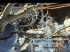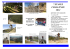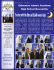# GRADED ALGEBRAS AND GALOIS EXTENSIONS

## Transcription

```Revista de la
Union Matematica Argentina
Volumen 25, 1971.
by D. J. Picco and M. -I. Platzeck
at
p~one~o~ Albe~to
Gonzalez Vomlnguez
We establish in this paper a connection between Galois extensions
of a commutative ring and algebras graded over the Galois group.
As in the case of Galois extensions whose group is Z2' we introduce a Brauer group associated to central separable algebras that
are not needed.
The methods of
produc~
, where Galois extensions ap -
pear as centralizers of the homogeneous component of degree 0 of
algebras graded over Z2' do not work for arbitrary G; here Galois
extensions appear as centralizers of the full graded algebra in a
new algebra, the construction of which from the given graduation
is similar to th& construction of a twisted group ring
from
an
,

action of a group.
After this, we use graduations to obtain the results of
on the cohomo10gica1 description of Galois extensions that have
normal basis.
1.
Let R be a commutative ring and G a
finite abelian group; we shall consider R-~lgebras graded over G,
that is R-a1gebras A with a decomposition A
~
A 's are R-submodu1es such that A .A
if a E A we write
a
a =
a
1:
11
C A
all
OEGAo where the
a(o) to denote the homogeneous components a(o) of a.
If A
and Bare G-graded we consider A ®R B as G-graded by A ® B
= eo(A® B)o with (A® B)o = ~a.s=o\'(® BS·
If A is G-graded, we denote with AE the R-a1gebra defined as fo110ws:.AE is the free left A-module generated by {eo: a E G} with
402
multiplication given by ae .be
a
n
sociative R-algebra with unit
a E A
the R-algebra monomorphism
a.b( a_l)e
n n ; then AE is an as
Iaea'
---+
We consider A CAE via
a.1 = La
\ ae a '.
then
~a·a = In a(a- 1 n)e n
LEMMA 1. Let A be a G-graded R-algebra.
R then AE is separable over R.
If A is separable over
Let m: A ® AO ---+ A be the multiplication map and
e E A® AO such that m(e)
and (l®aO-a® 1 0) . e = 0 for every a E A. If we write e
I a f a with f 0 E (A ® A0) a then m(f 1 )=
Proof·
and (1 ® aO - a 01°).f 1
0
for every a E A ; thus we assume e=£
(i.e. that we have a homogeneous splitting of
If
e=
e .
If A is a
phisms of
trivially
action of
L ea 0
eO E AE 0 AEo , then
a
e splits
m: A0 AO
---+
A)
AE ® AEo
---+
AE
G-graded R-algebra, we consider G as a group of automo
AE by the action given by a(ae ) = ae . Then G acts
n
an
on A C AE and if Z(A) is the centralizer of A in AE th
G in AE induces an action of G in Z(A).
Let us recall the following facts from (, Def. 4.5). If R is
a commutative ring, A a faithful R-algebra and G is a group of
automorphisms of A, then A is said to be a Galois extension of R
with group G if the following equivalent properties hold:
a)
AG = R , A is finitely generated and projective over Rand
the map AG ---+ EndR(A) is an isomorphism.
b)
AG = R
if F is the ring of functions from G to A then
f: A ® A ---+ F defined by f(a ® b)(a) = aa(b) is bijective.
Clearly b) is equivalent to b') AG = Rand f: A ® A
defined by f (a ® b) = I aa (b) a is bij ective.
---+
AG
a
Moreover a) is equivalent to a') AG = Rand AG is central separable over R. Indeed, if a) holds AG ~ EndR(A) is central separable over R; conversely, assume AG is central separable, then A
is finitely generated faithful projective over R and therefore
403
AG - - - 7 EndR(A) is injective (since it is the identity over R) ;
now it follows from  that the map is onto since the centralizer of its image is AG = R.
If G is a fixed finite abelian group and A , B are Galois extensions of R with group G, then A ® B is a Galois extension of R
with group G
x
G; as in  , we see that if H
=
{(cr,cr- 1) E G x G},
the (A ® B)H is a Galois extension of R~with group G
x
G/H
G
Clearly, if ER(G) denotes the set of G-isomorphisms classes of Ga
lois extensions of R with group G, then the above construction de
fines a product under which ER(G) becomes an abelian group (see
) whose identity element is E
Ell crEG R e cr with e.e
Ii
cr T]
crT] e cr
, the trivial Galois extension of R with group G (the
=
cre T] = e crT]
algebra of functions from G to R).
PROPOSITION 2.
Let A be a G-gpaded aentpaZ sepapabZe R-aZgebpa.
Then the aentpaZizep ZeAl of A in AE is a GaZois extension of R
with gpoup G.
Ppoof. Since A is central separable, we have (, Th. 3.1)
AE = A ® ZeAl and therefore zeAl is separable over R. On the
other side, if e E (A ® AO)I splits m: A ® AO - - - 7 A and we write
e = L
f. t
cr with tEA
cr
cr ® Acr -1
such that a(e).u cr
that x
=
Ecr
ucr~
=
then 1
f
u cr with u cr
Lcr
ucre.a(e) , Y a(e) E Ae .
Z(A)G
~
v
Then we can verify
ecr belongs to the center of ZeAl and EcrEG cr(x)
Therefore Z(A)G is separable over R (if e
~:
met") E Al
Z(A)G ® Z(A)G given by
= 1.
E.1a.1
®1
b~, then
~(a)
is a two sided Z(A)G-map that splits Z(A)G ® Z(A)G
~
Z(A)G) .
On the other side, Z(A)G = R and since the automorph.isms of ZeAl
are easily seen to be linearly independent over zeAl, we conclude
that the center of Z(A)G is R, i.e. Z(A)G is central separable
0-
ver R.
We define now a Brauer group BG(R).
If M
Ell
crEG cr
404
tion given by (EndR(M))
=
~
HomR(M ,M
ann
an
).
separable R-algebra is trivial if there exists a graded R-algebra
isomorphism
A.~
EndR(M) with M a finitely generated, faithful
pr~
If A and Bare G-graded central separa~
ble over R, A is equivalent to B if A ® TI
B ® T2 where TI ' T2
are trivial; then BG(R) is the set of equivalence classes of Ggraded central separable R-algebras under this equivalence; then
BG(R) is an abelian group in which the inverse of A is AO, since
the canonical isomorphism: A ® AO
---+
As in
, Prop. 5.3 , we can see that the class of A in BG(R) is the
identity if and only if A is trivial.
LEMMA 3.
If A E BG(R) is triviaZ then leA)
A = EndR(M) with M =
M ; let u a : M ---+ Ma be the cor
responding projection, then u.u
= 0
U
\' U
=
,u a E AI'
a n
a,n a ' L
a
Then I{J: E ---+ AE defined by l{J(e n ) = La u an e a -1 is an R-algebra m~
Proof·
~
a a
nomorphism such that I{J(E) C leA) and I{J: E
morphism; therefore I{J(E)
LEMMA 4.
Proof.
=
Let h: (A ® B)E
---+
leA) is a G-mono-
= E in EG(R).
leA) and leA)
If A , B E BG (R) then l (A ® B)
---+
=
l (A) . l (B) in EG (R) .
AE ® BE be the map defined by h(e,)
= Lan",
\'
e ~ e . then h is an R-algebra homemorphism
an'
h(a GIl b)
=
a ®b
thus h maps l(A ® B) into the centralizer of
A 8B in-AE ®BE, i.e., into leA) ® l(B).
\' -, e aCt ® e C! -1 n
L
an
h
Z(A GIl B)
---+
and
=
Since C! ®C!-l h(e,) =
h (e , ) , we have
(l(A) GIl Z(B))H
=
leA) • l(B)
and being
h
a G-
map we conclude that l (A ® B) = l (A) . l (B)!
LEMMA 5.
Let L be a GaZois extension of R with group G.
Then
405
there
e~ists
A E BG(R)
Let A
Proof·
=
s~ah
LG with A
La.
a
zeAl CAE and the map «I: AE
a l (1) E L (if a
«I
I Z(A)
=
Lah a a
that L
Z (A) .
Then LG E BG(R).
--+
Consider
L defined by «ICLaaae a )
E LG , a(1)
=
Lah a ).
We
show
that
is an antiau tomorphism of Galois extens ions.
Observe that La
h,e E LG.
La h.haa
U
a e a E zeAl implies h.u"
In particular, if u l
La haa(h)a
=
=
uah and e u a
=
uae-le,
La
h a with h E L we have
a
a
and therefore haa(h) = h.h a V a E G , h E L
=
\
u e
= \ h a
L.a a a ' v = La vaeaE ZeAl , u l
La
a
'
VI = La ha'a then ul·v l = La,n h a a(h')an
=
L
h'
h
an
and
theren
a,n n a
fore «I(u.v) - L
h' ha = «I(v) «I (u). On the other side, if
a,n n
u = La u a e a E ZeAl , u l = La h a a then for nEG we have n(u)
Let now u
=
La Un-lae a and since Un-l
nUl n- 1
La n (h a ) an- 1 (1) = La n (h a )
n«l(u) , i.e. «I: zeAl
,
«In (u)
=
Un-l (1)
--+
L is a G
map, and since «I is also an R-algebra homomorphism it follows
that «I is an isomorphism.
It follows from lemmas 3 and 4 that the correspondence A - - + ZeA
induces a group homomorphism Z: BG(R) - - + EG(R). Consider now
the map from the Brauer group B(R) of R (see ), to the Brauer
group BG(R) obtained by considering an ungraded central separable
algebra as trivially graded. We clearly obtain a group homomorphism
B(R) ~ BG(R).
PROPOSITION 6.
ian group.
Let R be a commutative ring and G a finite abel-
Then
is an split exact sequence.
Proof.
If A E B(R) and we grade i t trivially we have a.e a
ea.a
406
in AE for every a E A ; it follows that Z(A) = E.
~:
BG(R)
~
B(R) be the group homomorphism obtained considering
~IKer(Z)
to show that
that Z(A)
=
Let
E and A
Since
is injective.
~i
= identity, it suffices
Let then A E BG(R) be such
Since Z(A)
EndR(M).
=
E , Z(A)
with v.v
Lav a = 1 ,v a a(v l ). If vI = lf u~e~~
a n = 8 a,n v a
with u a E A we have v n = Laaa
u e -1 n = LU~n-1
e~
v .v
vv
vSince
an
= 8 a,n v a , it follows that u a .u = 8 a,n u a and Lava = 1 implies
n
1.
the
On
other
side,
=
since
va E Z(A) we have feua
Laua
v
v
uaefe for every f e e Ae. Let now be Ma = ua(M). Then M = EllaEGMa
and for fe E Ae we have fe(ua(m)) = (feua)(m) = (uaefe) (m)
uae(fe(m)) , i.e. fe(Ma) C Mae and therefore the graduation of
A is induced by the graduation M
=
Ell aEGMa of M ; thus A is triv-
ial in BG(R).
2.
ABELIAN EXTENSIONS WITH NORMAL BASIS.
Let G be a finite abelian group and E the trivial Galois extension of a commutative ring R with group G. The map EG ~ EG
defined by aa - > - a-1(a)a-1 is easily seen to be an R-algebra
antiautomorphism of EG; thus from the usual structure of left
EG ® EGo-module on EG we obtain a structure of left EG ® EG-module on EG, which is explicitly given by aa ® bn.x = aa.x.n~(b)n~.
Considering E as a left EG-module, we have that E ® E is a left
EG ® EG-module and the canonical map ~: E ®E - > - EG (induced by
~(e ® f) = L
ea(f)a) is an EG ® EG-isomorphism. Since
aEG
EG ® EG ~ EndR(E) ® EndR(E) ~ EndR(E ® E), it follows that R-auto
morphisms of E ® E and EG have the form x
->-
u.x
with
u E U(EG ® EG).
Let us recall the definition of Harrison's complex: the correspo~
dences alx •. • xa - + a l x •• • xa .xa .)(0. 1" .. . xa induce R-algebra
n
~
~
~+
n
.
407
homomorphisms 6 i : RG n ~ RG n+ l , i = 1, ... ,n. If
no ' 6 n+ l
are induce d by a l x ... xa n ~ 1 x a l " ... xan ' al" ... X.anx1
respec tively , then 6 i : U(RG n) ~ U(RG n+ l ) and Harris on's comple
x is
U(RG n ) , n ~ 1 with 0: U(RG n ) ---+ U(RG n+ l ) define d by
o(x)
=
6.(x)
<_l)i.
We
shall use the second cohomo logy group
l.
2
H (R,G) of this comple x; with the usual identi ficatio ns,
it is ob
tained from
TT
U(RG)
with o(x)
~
U(RG ® RG)
---+
U(RG ® RG ® RG)
x ® X.6 l (x- l ) , where 6 1 (L ar a a)
= Laraa ® a
if
X E U(RG) , o(x) = 1 ® X.6 2 (:X:).6 (X-1 ) x -1 ® 1 i f x
E U(RG ® RG),
1
where 61cL
a,n r a,n a®n )=l a,n r a,n a®a ®n,6 Cl
.a®n )=
2
a,n r a,n
= Lo,,,,To ,,,a ® n
® n
Let - : RG ® RG
---+
RG ® RG be the map induce d by
a ® n ---+ a ® an-I ; clearl y - is an R-alge
bra isomor phism of
RG ® RG, _2 = 1. Since RG ® RG C EG ® EG , then RG ®
RG acts on
E ® E and EG. Let us write u[xl , u(y) to denote the
corres ponding action of u E RG ® RG on x E E ® E , Y E EG. Then
it is
straig htforw ard to see that, for u E RG ® RG , o(u) =
1 if and
only i f
(1 )
where m: E ® E
E C EG as E.1.
u(m ute ® fl) = u(e) .u(f)
---+
'fe,fE E
E is the multip licatio n and we consid er
Let u E U(RG ® RG) with o(u) = 1 and let X = ~(E.a)
C EG; since
a
U E U(RG ® RG), x ---+ u(x) is an R-auto morphi
sm of EG and therefore EG=ED EG-X ;if u =l
r
a®
n
then
u(E.a)
= I
r
ClEas- 1
a
a
a,n a,n
CI,S CI,S
..
= I r
oClES-l
a
=
~(E)a
, and a~(en) = I r oaClenS- I = I r oClaenS-I=
Q,p
Q,p
Q.P
= ~(a(e)an). Now relatio n (1) shows that XI.X C Xl
I
and it follow s that X .X e x , i.e. we have a gradua
tion on EG;
.
a n
an
call [EGl u this graded algebr a and ~(u) the image of
= u(a e n)
408
[EGl u E BG(R) in EG(R) . We shall show that u
map H2(R,G)
---+
---+ ~(u)
induces a
EG(R)
Observe first the following : u and v define the same graduation
on EG if and only if v = u.t ® 1 with t E U(RG); in fact, if ~(E)
= veE) we have Ii-I.v(E) C E; if ~-I.v = I r 0,11 0 ® 11 then
1 EEfor every e EE implies r
Ir 0,11 0.e.ll.
0,11 = 0 ifo# 11; thus
Ii-l.v = I o r 0,0 0 ® 0 = 1I1(t) , t = I a ro,a
o E U(RG) and therefore
v = u.1I 1 (t) = u.t® 1; the converse is clear.
Let now u, v E U(RG ® RG) be
=t®1.1®t.1I1(t
-1
).u
~ocycles
such that V= o(t).u =
-
then v = 1I1(t).1 ®t*.t
-1-
®l.u,where
t* = I 0
r 0- 10
if t 0
= Ir 0
Since lI(t) (Eo)
Eo we have v(Eo) =
= t- l ® 1.1 ® t*.~.lIdt)(Eo) = t- l ® 1.1 ® t*(~(Eo)) . On the
other side, t- l , t* EU(RG) C RG C EG and it is clear that
(t- l ® 1.1 ® t*) (x) = t-l.x.t* for x E EG; thus v(Eo) =
= t-l.u(Eo).t, which shows that [EGlu
and [EGl v are isomorphic
as graded algebras and therefore that they have the same image
in EG (R) . It follows that we have a map 'I' : H2 (R,G)
Let uE U(RG ®RG) be acocycle. Since EG =e 0
tion on EG, ~(E) is an R-subalgebra of EG; note that u(Eo) = u(E)o
implies 0 E ~(E)
and therefore o.~(E).o-l C ~(E) ; this relation
allows us to define an action of G on ~(E)
since ~(o(e)) =
• o~(e)o-l , ~(E) is RG-isomorphic to E; moreover, if we consider
the twisted group ring of u(E) with respect to that action of G,
we have a
map u(E)G
---+
• u(e)o(u(f))Ol1 shows that
EG and ~(e)o . U(f)l1
u(E)G ~ EG and by lemma 5 we have
,!,(u) = u(E) ; in particular this shows that
sis, i. e.,
'1':
H2 (R,G)
---+ \ ;
'I'(u) has a normal ba-
(R) , the subgroup of EG (R) formed
by algebras with normal basis. Moreover, it follows from relation
(1) that 'I'(ti) = E with the usual action of G and product defined
409
by (e,f)
~ m u [e ® f l . This remark can
be used to show that
2
0/: H (R,G) ~ AG(R) is a group homom
orphism ; indeed , if H
={ (a, a-I) : a E G} then j
E ---+ E ® E define d by j (e ) =
a
I a e aa ® e - I maps E isomo rphica lly onto E ® EH, and
j a = a ® 1. J.
ex.
Then we can verify that j is an isomop hisrn from o/(uv)
ontoo/ (u)o/(v ).
Let us finall y show that
0/:
H2 (R,G) ~ AG(R) is an isomor phism.
If o/(u) is trivia l, from EG ~ ~(E)G ~ [EGl
u f it follow s that
there exist an R-alge bra isomor phism w: EG ~ EG
such that
w(a) =a and weE) = tilE) ; we know that w must
be of the form
x
~
w(x) with wEU(c G ® EG) and since w(a) =a we have
wEU(RG ® RG) ; then ~-1w(E) CE and we have ti-1w =llJ(t)
with
tEU(R G).
Let us show that u = "l (t- 1 ) ; to this end, we can
assume R to be
local ; then w is an inner automo rphism of EG, i.e.
w(x) = p.x.p- 1
with p E U(EG) ; but w(a) = a implie s p EU(RG)
Then w = p ® p*-1
and u = ~(lll(t-l)) = lldp). l ®p-1. t -1® 1; now
6(lldp ).1 ® p-1) 6(t-1 ® 1) = 6(p ® 1)6(t- 1 ® 1)
1 = 6(u)
implie s
1 ® p.lll (p-1) ~ 1 ® t.ll (t- 1 ) and theref ore u = "l(t). -1 "" -1
t
~ t
.
1
Thus 0/ is inject ive.
Let now LEE·G(R) ,then there exists an RG-iso morphi
sm j:E ~ L
and if we denote with J the R-alge bra isomor phism define
d by
LG ~ EndR(L ) ~ EhdR(E ) ~ EG , then J : LG ~ EG is
such that
J(a)
EG =
= a ; then settin g Xa
~aXa
J(Aa) we obtain a gradua tion on EG,
whose image in EG(R) is L.
the map define d by f (e)
J (j (e)
a)
Let now f : EG
---+
EG be
; then f is an R-auton ,o'rphis m
of EG such that flEa) = Xa ; thus there exists z EU(EG
® EG) such
that z(E a ) = Xa
note that f(a.x.n ) = af(x)n and theref ore
z EU(RG ® RG). I f z = I
r
a ® n,let t=I (I r
a, n a, n
a n a, n)aEU(RG) and
410
set v
'=
ill
(t -1 ) . 1 ® t. -z . From the relation Xl,X l
C
Xl we have
z(E).z(E) C z(E) , i.e. z-l(z(E)z(E)) C E and it fo11ows that
z (m
vI
e ® f])
z(e).z(f)
Now; = t- 1 ® t*. z and since t- l ® t* (x)
have ~-l(~(e).v(f))
=
t-l.x. t i f x
E
EG, we
z-l(z(e).z(f)) and therefore v(m v Ie ® f])
=;(e).~(f)j i.e. v is a cocycle. Moreover, v
=
t- l ® t*. z implies
-
v(Eo) = t -1 ® t*(z(Eo)) = t- 1 .Xo.t , i.e. EG with the graduation
EG = moxo is isomorphic to [EG}v and therefore L
= ~(v).
Thus, we
have obtained the following:
If u E U(RG ® RG) is a eoeyeZe in the Harrison's
PROPOSITION 7.
compl~.
Zet
E be the R-aZgebra obtained defining a produet in
u
E by (e,f) ---+ mule ® f] . Then with respeet to the standard
aetion of G on E, G is a group of R-aZgebra automorphisms of Eu
and Eu is a GaZois extension of R with group G. Moreover,u ---+Eu
defines a group isomorphism H2 (R,G) ~ AG (R)
3.
ABELIAN EXTENSIONS OF FIELDS.
We shall show now that for certain fields Harrison's cohomology
can be replaced by the usual cohomology in order to study Abelian
extensions (note that Galois extensions of fields have normal basis). Let K be a field and G an arbitrary group. We shall consider now central separable algebras with a representation of G as a
group of Kc~lgebra automorphisms of A. If M is a KG-module then G
acts on EndK(M) by o(f) = ofo- l ; call a G-algebra A trivial if
there is a G-isomorphism of K-algebras A ~ EndK(M) for M a KG-mo~
ule. If A and Bare G-algebras, let G act on A ® B via o(a ® b) =
= a(a) ® o(b), a E A, b E B. If A and B are central separable Galgebras, then A is equivalent to B if there is a G-isomorphism
A ® TIS! B ® T2 with Tl' T2 trivial G-algebras ; we obtain in
411
this wayan equivalence relation in the set of G-isomorphism class
es of central separable G-algebras; if B~ (K) is the quotient set
then
B~
(K) is an abelian group (note that the inverse of A E Bd (K)
is A0 , since A ® A0
-+
En'\z (A) is a G- isomorphism) .
Let A be a central separable G-algebra. If a E G, then a is an inner automorphism of A, i.e. o(x)
Since aT] (x)
=
aa x a-I
for some unit a o E A.
a
(aa a T] ).x.(aa a T] )-1 for every x EA, we have aOaT]
= k
a
with k
E K* . If we consider G acting trivially on K,
0,11 all
0,11
then k
is a 2-cocycle of G with coefficients in K . If o(x)
O,T]
-1
= b xb- 1
and b b = k' b
, then k0,T] = k'0,11 ka k11 kall with ko E K
O,T] all
a a
a 11
such that b a = ka a a and therefore the cohomology class of k a ,T] in
=
H2 (G,K) is well defined by the action of G in A
We can now verify that the product of the cocycles associated to
algebras A and B is the cocycle associated to A ® B ; if A =
= EndK(M) with MaG-module then o(x) = aoxa~1 with a o E EndK(M)
and therefore the cohomology class associated
all
to A is trivial. It follows that the correspondence A - + ko ,11
such that a a
a 11
a
defines a group homomorphism B~(K) ~ H 2 (G,K*) . Consider now
B(K)
---+
B~(K)
obtained by letting G act trivially on central
separable algebras .
PROPOSITION
7.
Let K be a field and G a finite group. Then
is an split exact sequence.
Proof.
We first show B' (K)
G
-+
H2 (G,K*) is onto. Given
} E H2 (G,K*), let E be the trivial Galois extension of K
0,11
with group G and let A be the cross product associated to E and
{k
k
0,11
; explici tely A
=
EDoEGEU o wi th u au 11 = k 0,11 u aT]' u a e
=
o(e) u 0'.
412
then A E B(K) and G acts on A by a ( x)
=
uaXU -1
a ; clearly the cocy-
cle corresporiding to this action is {k
B~(K)
phism
~
Clearly B(K)
}. Consider the homomora,1l
B(K) obtained by forgetting the action of G.
~
B~(K)
~
B(K) is the identity; to complete
the proof we need only show that the restriction of e to
Ker(B~(K)
k a,
T) \
a
Il
B(K)) is injective; let then A E BG(K) be such that
is trivial and A
by a(x)
a a
~
=
= a all
=
EndK(M) . If the action of G on A is given
a a xa-a 1 we can assume (since k a,1l is a coboundary) that
. since a E EndK(M) this means that M is a G-module
'
a
and that the induced action of G on EndK(M) is the given one on A.
Thus A is trivial in
B~(K).
Assume now that G is a finite abelian group of exponent e and K is
a field of characteristic prime to e that contains the e-th roots
of unity. If G is the group of characters from G to K, we know
A
A
that E ~ KG; indeed T: KG ~ E defined by T(x)
=
La EG
x(a)e a
is a K-algebra isomorphism with inverse T- 1 : E ~ KG given by
1
T- (e a )
=
lin (Lx E G x (a
-1
) x) (see [41) . If A is a K-module, to
say that A -- e aEG Aa
where the Aa 's are K-submodules of A is equivalent to say that A is a module over E, also equivalent to say
A
that A is a KG-module, as follows from E
A
ecr E GA a
is
KG
If a decomposition
related to an action of G on A by the isomorphism
A
E ~ KG we can see that x(a)
a(a) E Aa and Aa
~
=
{
=
LaEG
a E A : x(a)
-1
x(a
x(a
)a(a) i f a
- 1
)a IJx. E G}
in particu-
e aEG
A
a
on A if and only if the related action of G on A is an action by
lar, if A is a K-algebra then A
K-algebra automorphisms of A. Note that for a graduation A
=
A and an action of G on A related as above, the isomor= e a EGa
phism KG
T : AG
~
~
E can be extended to a K-algebra isomorphism
AE with T(a)
= a for a E A.
413
Clearly trivial G-graded algebras are related to trivial G-algebras;
if A and Bare K-algebras, the product graduation of A ® B is related to the product G-structure of A ® B and it follows that
BeCK)
~
,
Be(K} canonically, which in turn implies that Ee(K)
~
~ H2 (G,K*).
Let us explicitate the isomorphism H2 (G,K*)
-+
EeCK) : i f
~} E H2(a,K~ let as in proposition 7, A = @ - Eu with u u =
x, '"
xEe
x
x 1/!
= k ~u~, u e = x(e)u . Then
acts on A by x(x) = u .x.u- 1 ; for
x,'" x'"
x
x
x
x
the G-graduation on A related to that action of G on A we have a
{k
a
-
K-algebra isomorphism T: AG
- + ~E
such that T(a)
a and if we
=
consider the action of G on AE, we obtain an action of G on AG;
A
thus ZeAl is isomorphic to the centralizer of A in AG, which is
easily seen to be
e XEG-
K.u-Ix with a(u-Ix) = x(a-l)u-1x.
X
X
Thus
X
the Galois extension of K associated to {k
,,) is the K-vector
k- 1 a
and G
space L = e XEG K ax ' with product given by a x ·a1/!
x,1/! xw
acting as a(a ) = x(a- I ) a
x
x,'"
x
Let G be a finite abelian group of exponent e and K a field whose
characteristic is prime to e. Let R be the field obtained adjoi~
ing the e-th roots of unity to K and W the Galois Jroup of Rover
K. If G is the group of characters from G to R, we shall see
that Ee(K) ~ H~(G,R*) (see  Cor. 4.8, Th. 2.2), where the coh£
mology groups Hw(G,K*) are defined by W-invariant cochains, i.e.
such that wf(xI' ... 'X n )
= f(wxI' ... 'wxn).
Observe first that if
[ is a Galois extension of R such that W can be extended to a
group of automorphisms of
[,
then [
[w ® R·, moreover, i f the ac
tions of G and W commute on [, then G acts on [w and [w is a Galois extension of K with group G, as follows from LG
=
LWG ® R
414
If ~ x,ljJ E R* is a W-invariant cocycle, let [
m R a~"- the extension of K associated to it, that is, ax·a.
~;!ljJaXljJ ,o(a x )
then the relation w(k ~) = k
implies that dex
x,,,,
wx,wljJ
fining w(a x ) = awx for w EW, we extend W to a group of automor= x(o-l)a
phisms of I which obviously commute with G and then L = i W EEG (K).
We can verify that the correspondence k
- + - L induces a group
x,ljJ
-W
homomorphism ~ (G , K*) --->- EG (K) . If L is trivial, then L = L
has a normal basis genera ted by a E L such that
= 6
a (a);
X
=
L0 kXO
11
(a) =
(a) with kO E K, we deduce that
x
w(k')
=
k'
and
k'
=
k
k'
k'
which
shows
that
X
wx
xljJ
x,ljJ X 1jJ ,
H~(G,K*) - - - + EG(K) is injective.
0,11
writing a
o (a)
0
Let L E EG (K) and L = L ® K; if A = LG , A
LG = A ® K then L
and L are the centralizers of A and A in AE and AE respectively.
Consider the action of G on A related to the graduation of A and
let T: AG
--+
AE be the canonical isomorphism.
W acts on AE; it is clear that T: AG
if W acts on AG by means of w(ax)
L ~ T-1(Z(A)) = T-1CZ{A))W.
ing to A in H2 CG,K*).
Let a
---+
Since AE = AE ® K,
AE is a W-isomorphism
1 ® weal .wx
Then
Let us determine a cocycle correspon~
L uoe o
E ZeAl generate a normal
basis of ZeAl and ~ = r-l(a) E AG; then
e = L a xx
wi th
a
x =
= l/n Lox(a-l)u o ; note that x(a).a x = ax.a ; if b x is a unit of A
such that x(a) = b x a b~l we must have ax E K.b x and since
L axx
generates a normal basis, ax -I 0 and therefore ax is a unit of A;
then xCa)a x
ax a
shows that a cocycle corresponding to A can
be defined by the relation a xaljJ =
kX,ljJa xljJ .
Since w(a x ) = a wx '
and a wX.W'l',I, = a wxljJ , it follows that wCk x, "~,)
k wX,w",,J,' Now
'I'
T-ICZCA)), the centralizer of A in AG, is m K a~lx. If a~lx=ax'
we have then a .a", = k- l ",; ,I, and w(a)
a
and since
I
x 'I'
x,'/' x'"
X
wx
L = T-JCzeA)) = T-ICZ(A))W • we conclude that L is the image of
{k X,ljJ}
E H~(G,K*).
415
REFERENCES

M. AUSLANDER and O. GOLDMAN, The B~aue~ g~oup 06 a commuta-
tive
~ing,
Transactions of the A.M.S., Vol. 97 (1960), p.p.
367-409.

H. BASS,
Topie~
in
atgeb~aie
K-theo~y.
Tata Institute of
Fundamental Research, Bombay (1966).

S. CHASE, D. HARRISON and A. ROSENBERG, Gatoi~ theo~y and
Gatoi~
cohomotogy 06 eommutative
~ing~,
Mem. Amer. Math.
Soc. N°. 52 (1965), p.p. 15-33.

S. CHASE and A. ROSENBERG, A theo~em 06 Ha~~i~on, Kumme~
theo~y
and
Gatoi~
atgeb~a~,
Nagoya Math. Journal, Vol. 27
(1966), p.p. 663-685.

M. ORZECH, A eohomotogieat de~e~iption 06 abetian Gatoi~
exten~ion~,
Transactions of the A.M.S., Vol. 137 (1969),
p.p. 481-499.


B. VAN DER WAERDEN,
Atgeb~a, Vol. 2, 5 th edition, Springer-
Verlag.

C.T.C. WALL,
B~aue~ g~oup~,
J. Crelle, Band 213
(1964), p.p. 187-199.

P. WOLF,
Atgeb~ai~ehe Theo~le
de~ Gatoi~ehen Atgeb~en,
Deutschen Verlag der Wissenschaften, Berlin 1965.
Argentina
Recibido en agosto de 1971.
```

### Description and results - Mathematics Research Unit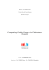### Acervo de la BIblioteca Jurídica Virtual www.juridicas.unam.mx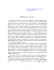### Évariste Galois, a Tragic Romantic Hero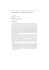### Gaming Technology in the Classroom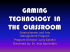### Muttonbird Panui (Flyer) 2014### NOTES ON GALOIS MODULES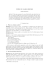### report of WMAAG 2015 - CIIT Lahore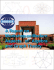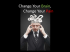### The Space Catalog Mission and Conjunction Analysis